# CBSE Sample Paper Class 11 – Chemistry (Term 2 Solution)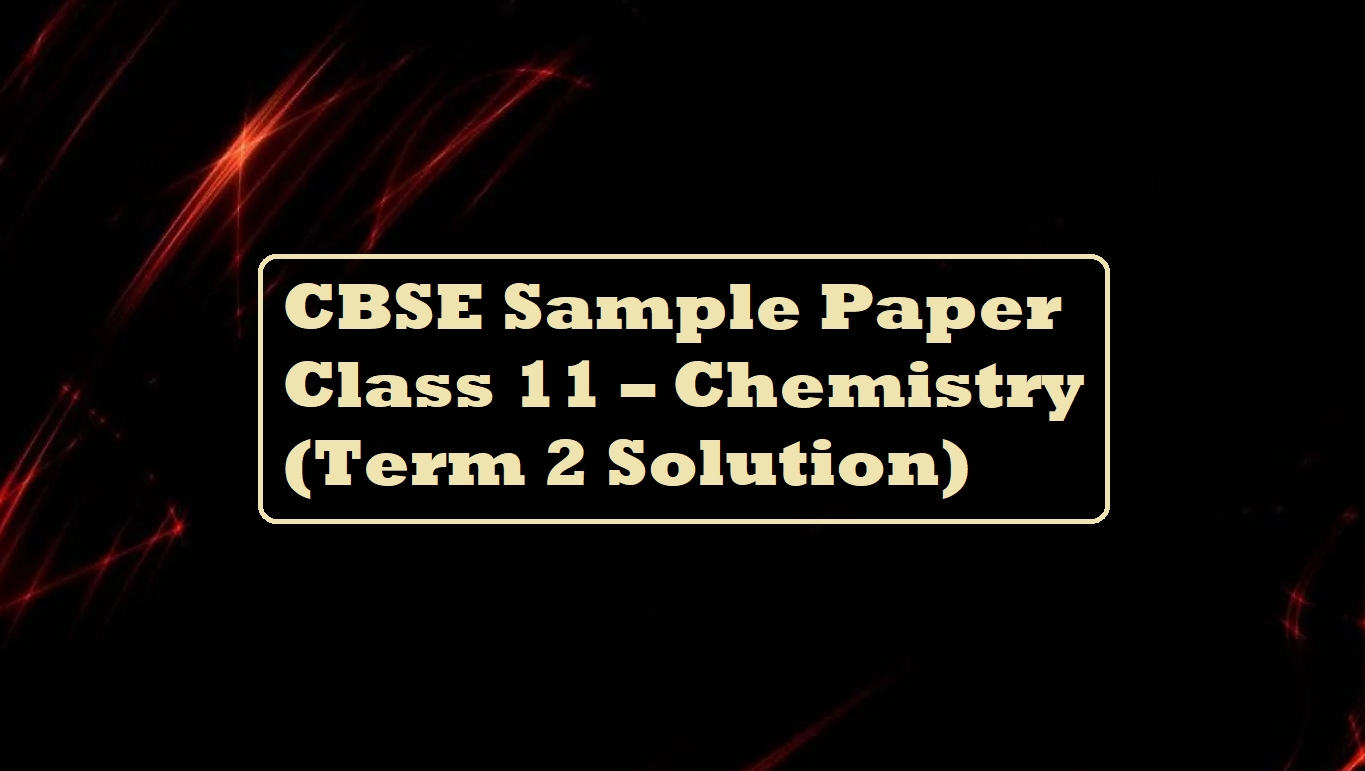# CBSE Sample Paper Class 11 – Chemistry (Term 2 Solution)

CBSE Sample Paper Class 11 – Chemistry (Term 2 Solution)

# To get paper click     —-     CBSE Sample Paper Class 11 – Chemistry (Term 2)

SECTION –A

Ans 1. (i) Dispersion force
(ii) Dipole-dipole interaction
(iii) Hydrogen bond
(iv) Dipole-induced dipole interaction

Ans 2. We have to find Density of ammonia (NH3)
T = 30 + 273 = 303K,
P = 5 bar, Mass of NH3 = 17gmol-1
R = 0.083 barLK-1mol-1
d = PM/RT,
d = (5 × 17)/0.083 × 303 = 3.379 gcm-3

Ans 3. (i) Arrange the following in increasing order of their hydration enthalpy.
Rb+ < K+ < Na+ < Li+,
(ii) Complete the following reaction equation
Na(s) + H2O(l) → NaOH(aq) + H2(g)

Ans 4. (i) If the pressure ′P′ of moist gas is given at temperature T, then to get the pressure of dry gas, the correction term which needs to be applied to the total pressure to obtain a pressure of dry gas is
PDry Gas​   = PTotal​ – Aqueous Tension
(ii) At a particular temp vapour pressure of acetone is less than that of ether because intermolecular forces of attraction are stronger in acetone than that in ether. Acetone has hydrogen bonding. Thud intermolecular forces of attraction between molecules of acetone are stronger.

Ans 5. Given,
Amount of the gas, n = 4.0 mol
The volume of the gas, V = 5 dm3
The pressure of the gas, p = 3.32 bar
R = 0.083 bar dm3 K–1 mol–1
From the ideal gas equation, we get
pV = nRT
⇒ T = pV / nR
= (3.32 × 5) / (4 × 0.083) = 50K

Ans 6. (i) Hydration enthalpy of Li is greatest
(ii) Stability of LiH is highest

SECTION –B

Ans 7. Conditions when a reaction be spontaneous
(i) Both ∆H and ∆S are positive and T∆S >∆H
(ii) Both ∆H and ∆S are negative and T∆S <∆H
(iii) ∆H is negative and ∆S is positive then the reaction is always spontaneous at any temperature.

Ans 8. (i) According to Hess’s law the enthalpy change for a particular chemical reaction remains the same whether that reaction takes place in one step or in multiple steps.
For Example: Let us consider the formation of CO2 from C and O2. The carbon might be burnt directly to CO2. On the other hand, carbon might be burnt to carbon monoxide and then the latter burnt to carbon dioxide.
C (s) + O2 (g) → CO2 (g) ΔH1 = 393.5 KJ
Step 1: C(s) + 1/2O2(g) → CO ΔH2 = -111 kJ/mol
Step 2: CO(g) + 1/2O2(g) → CO2 ΔH3 = -282 kJ/mol
_________________________
Overall change: C(s) + O2(g) → CO2 ΔH2+3 = -393 kJ/mol = ΔH1
(ii) (a) Evaporation of liquid water into steam is endothermic, so ∆H will be +ve
(b) Freezing of liquid water into ice is exothermic so ∆H will be -ve.

Ans 9. (i) As ionic size increases lattice enthalpy decreases much more than hydration enthalpy due to this solubility of alkaline earth metal hydroxide in water increases down the group.
(ii) Due to the smaller size of Li+ the hydration enthalpy of lithium cation is much more negative and E0Li+/Li is most negative, by the joint effect of ∆subH, ∆IEH, and ∆hydH lithium is a stronger reducing agent than sodium.
(iii) Due to very low first ionization enthalpy, alkali metals are highly reactive in nature due to these alkali metals not being found in Free State in nature.

Ans 10. (i) Due to the inert pair effect lower oxidation state is more stable in heavier elements in the p block, that’s why BCl3 is more stable than TlCl3.
(ii) BF3 behaves as Lewis acid because it is an electron-deficient compound (incomplete octet).
(iii) Due to the poor shielding effect 3d subshell electrons in gallium, the atomic radius of gallium is less than the atomic radius of aluminum.

Ans 11. According to the question, element A is Carbon, its monoxide (B) is CO, and dioxide (C) is CO2.
C(s) + 1/2O2(g) → CO(g),
C(s) +  O2(g) → CO2(g),
Fe2O3(s)  + 3CO(g) → 2Fe(s) + 3CO2(g)
OR
(i) PbO2 < SnO2 < GeO2 < SiO2 < CO2 (increasing order of acidic strength)
(ii) Sn-Sn < Ge-Ge < Si-Si < C-C (Increasing order of bond enthalpy)
(iii) C > Si > Ge > Sn > Pb (Decreasing order of first ionization enthalpy)

Ans 12. (i) IUPAC name of compounds CH3-CH=CH-CH2CH3 is pent-2-ene
(ii) There are two possible geometrical isomers of C4H8,
cis-butene-2 and trans-but-2-ene
(iii)  n-pentane and 2,2-dimethylpropane

Ans 13. (i) When Ethyne passed through red hot iron tube Benzene formed
(ii) Ethene is allowed to react with ozone and hydrolyzed in presence of zinc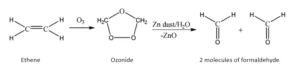(iii) Benzene reacts with conc-HNO3 in presence of concentrate H2SO4CBSE Sample Paper Class 11 – Chemistry (Term 2 Solution)

Ans 14. (i) Ethyne can be converted into ethanal by the reaction of ethyne with 40% H2​SO4​ and 1% HgSO4​. In this reaction, the hydration of the ethyne group takes place followed by the tautomerism to form ethanal as a product.
(ii) Propene to 1-propanol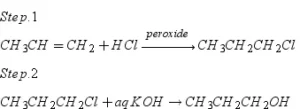(iii) Benzene to acetophenoneAns 15. H2 + Br2 ​→ 2 HBr
ΔH = Bond energy data for formation of bond  bond energy data for dissociation of bond
= − [2 × eHBr​] + [eHH ​+ eBrBr​]
= −2 × 368 + 435 + 192 = −109 kJ

Ans 16. (i) The standard entropy is not zero because there is always randomness in the system except at the absolute zero temperature, but the standard enthalpy of formation is the heat change when the compound is formed from its elements. As the elementary substance is naturally formed from its constituent elements, no heat is required and hence its value is taken as zero.
(ii) A process that can take place of its own or upon initiation under some condition. eg. Common salt dissolves in water of its own.

Ans 17. (i) Due to the high lattice enthalpy of LiF as compared to LiCl, LiF is almost insoluble in water. LiCl is soluble in acetone also due to larger anion as compared to cation, polarization occurs and thus LiCl possesses some covalent character.
(ii) Lithium is the smallest in size among the alkali metals. Hence, Lit ion can polarize water molecules more easily than other alkali metals. As a result, water molecules get attached to lithium salts as water of crystallization.

Ans 18. (i) Due to the inert pair effect Pb2+ is more stable than Pb4+. Whereas Sn4+ is more stable than Sn2+.
(ii) 3Ga+ → 2Ga + Ga3+
This is because Ga3+ is more stable than Ga+.

Ans 19. (a) Boron halides are electron deficient in nature so they form additional compounds with electron-rich species like ammonia and amines.
(b) (i) Anhydrous AlCl3 is used as a catalyst because it acts as a Lewis acid that can accept electrons by forming intermediates and also by speeding up the reaction. It also leads to the creation of carbocation which is used in the electrophilic substitution reaction.
(ii) The stability of the +3 oxidation state of group 13 elements decreases down the group due to the inert pair effect on ns2 electrons. This is because of the poor shielding effect of electrons in d and f orbitals.

CBSE Sample Paper Class 11 – Chemistry (Term 2 Solution)

Ans 20. Kolbe Electrolysis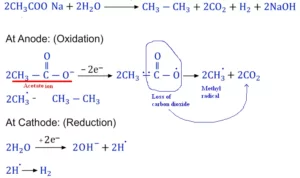Ans 21. (i) Ethene be prepared from Ethanol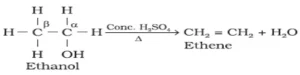(ii) Ethene be prepared from Ethyl bromide
CH3CH2Br + KOH (alc) → H2C = CH2 + KBr + H2O.

Ans 22. (i) Greater the s-character of a hybridized carbon atom, the greater will be its electronegativity. The s-character of the sp-hybridized carbon atom of acetylene is greater than that of the sp2-hybridized carbon atom of ethylene. So electronegativity of the carbon atom of acetylene (CH≡CH) is greater than the carbon atom of ethylene (CH2 = CH2).
The tendency of the H-atom attached to the more electronegative carbon atom to be removed as proton (H+) is relatively higher. So, the acidity of ethylene is less than that of acetylene i.e., acetylene is more acidic than ethylene.
(ii) Bond length order: Triple bond < Double bond < Single bond. In general, increasing order of bond length is therefore, C2H2 < C2H4 < C2H6

SECTION – C

Ans 23. (i) At a certain temperature  Kp = 9/4 for the reaction
CO(g)           +         H2O(g)           ⇌      CO2(g)           +        H2(g)
initial conc             10 moll-1                 10 moll-1                   10 moll-1                      10 molL-1
at equilibrium      (10 –  x) moll-1    (10 – x) moll-1       (10 + x) moll-1       (10 + x) molL-1
we know that  Kp = Kc (RT)∆n  and ∆n = 0,
then Kp = Kc = 9/4
now   Kc = [CO2][H2]/ [CO][H2O]
9/4 = (10+x)2 /(10-x)2
3/2 = (10+x) / (10-x)
X = 2,
Conc. of hydrogen at equilibrium = (10+x) = 10+2 = 12 molL-1
(ii) Kc will remain constant, it changes only with change in temperature.
(iii) Since the value of Kc is so high means the concentration of products is much larger in the system.
(iv) K2 = 1/
K2 = 1/
K2 = 1/7

CBSE Sample Paper Class 11 – Chemistry (Term 2 Solution)

To get Question paper click — CBSE Sample Paper Class 11 – Chemistry (Term 2)